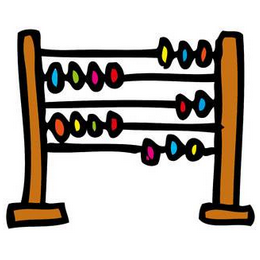# 文本挖掘实战：看看国外人们在病毒隔离期间都在家里做什么？

## 数据获取和预处理

``````tweet_words <- tweets %>%
select(id,
screenName,
text,
created) %>%
mutate(created_date = as.POSIXct(created, format="%m/%d/%Y %H")) %>%
mutate(text = replace_non_ascii(text, replacement = "", remove.nonconverted = TRUE)) %>%
mutate(text = str_replace_all(text, regex("@\\w+"),"" )) %>%
mutate(text = str_replace_all(text, regex("[[:punct:]]"),"" )) %>%
mutate(text = str_replace_all(text, regex("http\\w+"),"" )) %>%
unnest_tokens(word, text)``````

``````#Remove stop words
my_stop_words <- tibble(
word = c(
"https","t.co","rt","amp","rstats","gt",
"cent","aaya","ia","aayaa","aayaaaayaa","aaaya"
),
lexicon = "txxxxr"
)#Prepare stop words tibble
all_stop_words <- stop_words %>%
bind_rows(my_stop_words)#Remove numbers
suppressWarnings({
no_numbers <- tweet_words %>%
filter(is.na(as.numeric(word)))
})#Anti-join the stop words and tweets tibbles
no_stop_words <- no_numbers %>%
anti_join(all_stop_words, by = "word")``````

``````tibble(total_words = nrow(tweet_words),
after_cleanup = nrow(no_stop_words)
)``````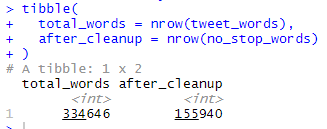## 词频分析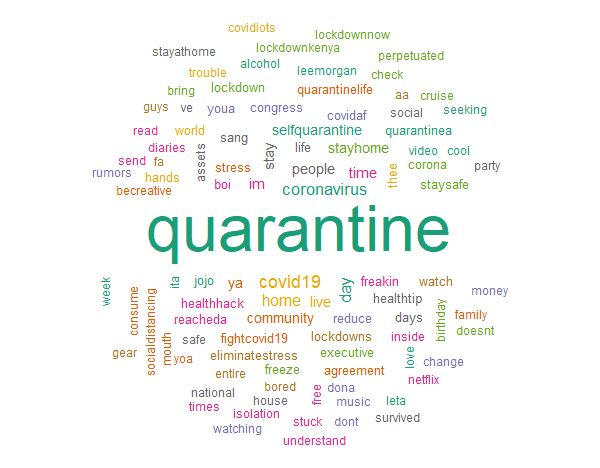`````` 隔离-出现13358次
Covid19 –出现1628次
冠状病毒-出现了1566次
天-出现1200次
家-出现了1122次``````

``````#Unigram word cloud
no_stop_words %>%
count(word) %>%
with(wordcloud(word, n, max.words = 100, random.order = FALSE,scale=c(4,0.7),
colors=brewer.pal(8, "Dark2"),random.color = TRUE))``````

## 最常见的正面和负面词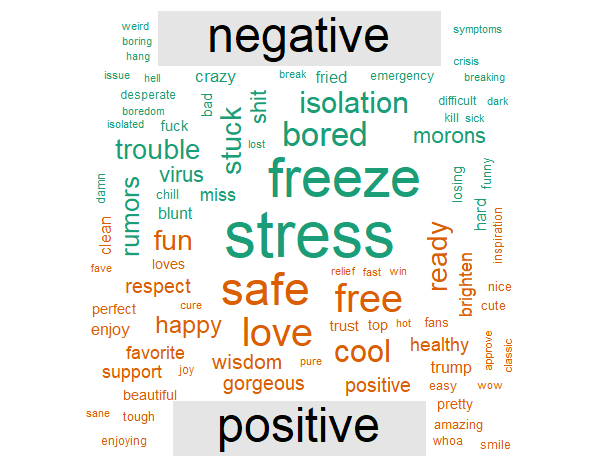``````#Positive and negative terms word cloud
no_stop_words %>%
inner_join(get_sentiments("bing"), by = c("word" = "word")) %>%
count(word, sentiment, sort = TRUE) %>%
acast(word ~ sentiment, value.var = "n", fill = 0) %>%
comparison.cloud(colors = brewer.pal(2, "Dark2"),
max.words = 100)``````

## 情感分析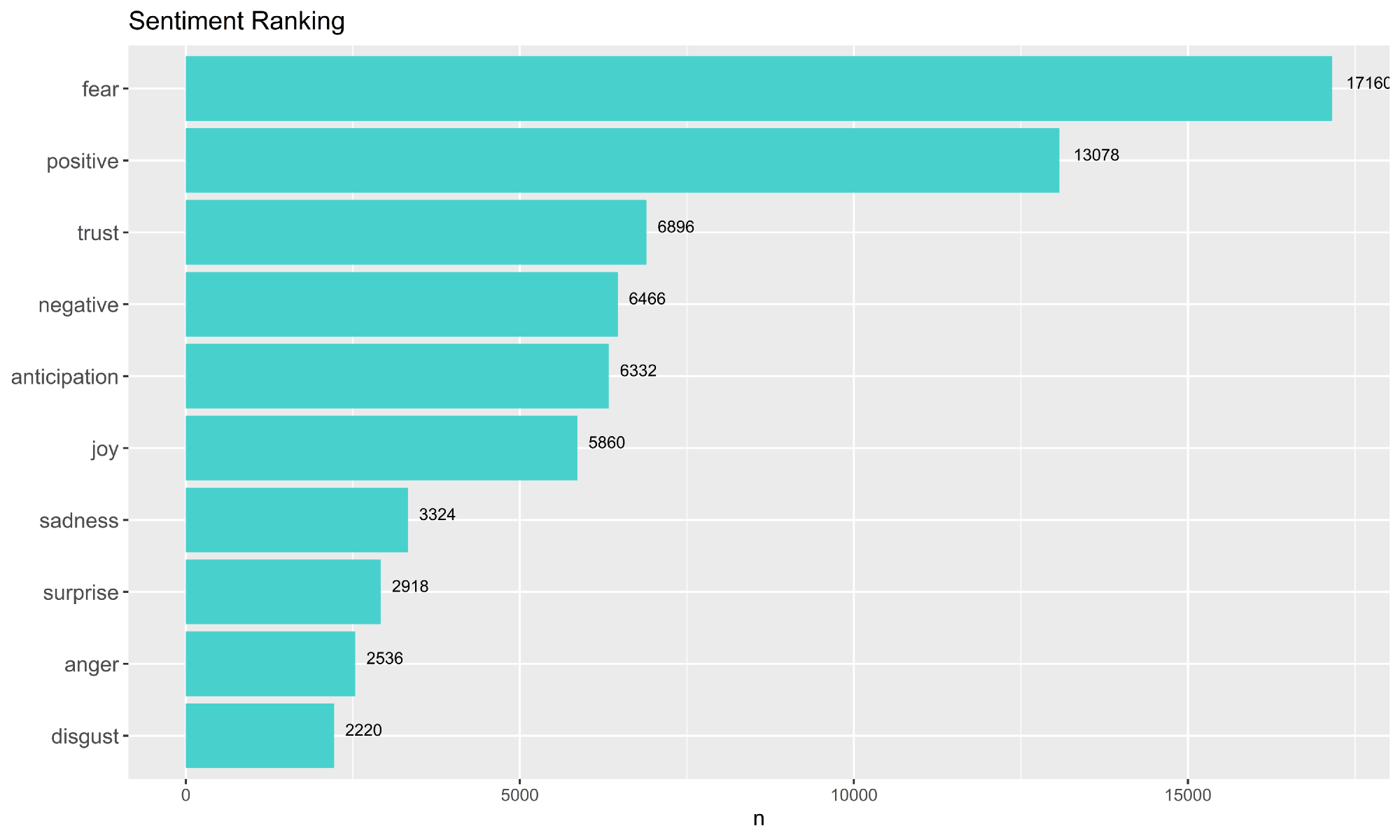``````#Sentiment ranking
nrc_words <- no_stop_words %>%
inner_join(get_sentiments("nrc"), by = "word")sentiments_rank <- nrc_words %>%
group_by(sentiment) %>%
tally %>%
arrange(desc(n))#ggplot
sentiments_rank %>%
#count(sentiment, sort = TRUE) %>%
#filter(n > 700) %>%
mutate(sentiment = reorder(sentiment, n)) %>%
ggplot(aes(sentiment, n)) +
geom_col(fill = "mediumturquoise") +
xlab(NULL) +
coord_flip() +
ggtitle("Sentiment Ranking") +
geom_text(aes(x = sentiment, label = n), vjust = 0, hjust = -0.3, size = 3)``````

## 情感内省-弄清人们的情感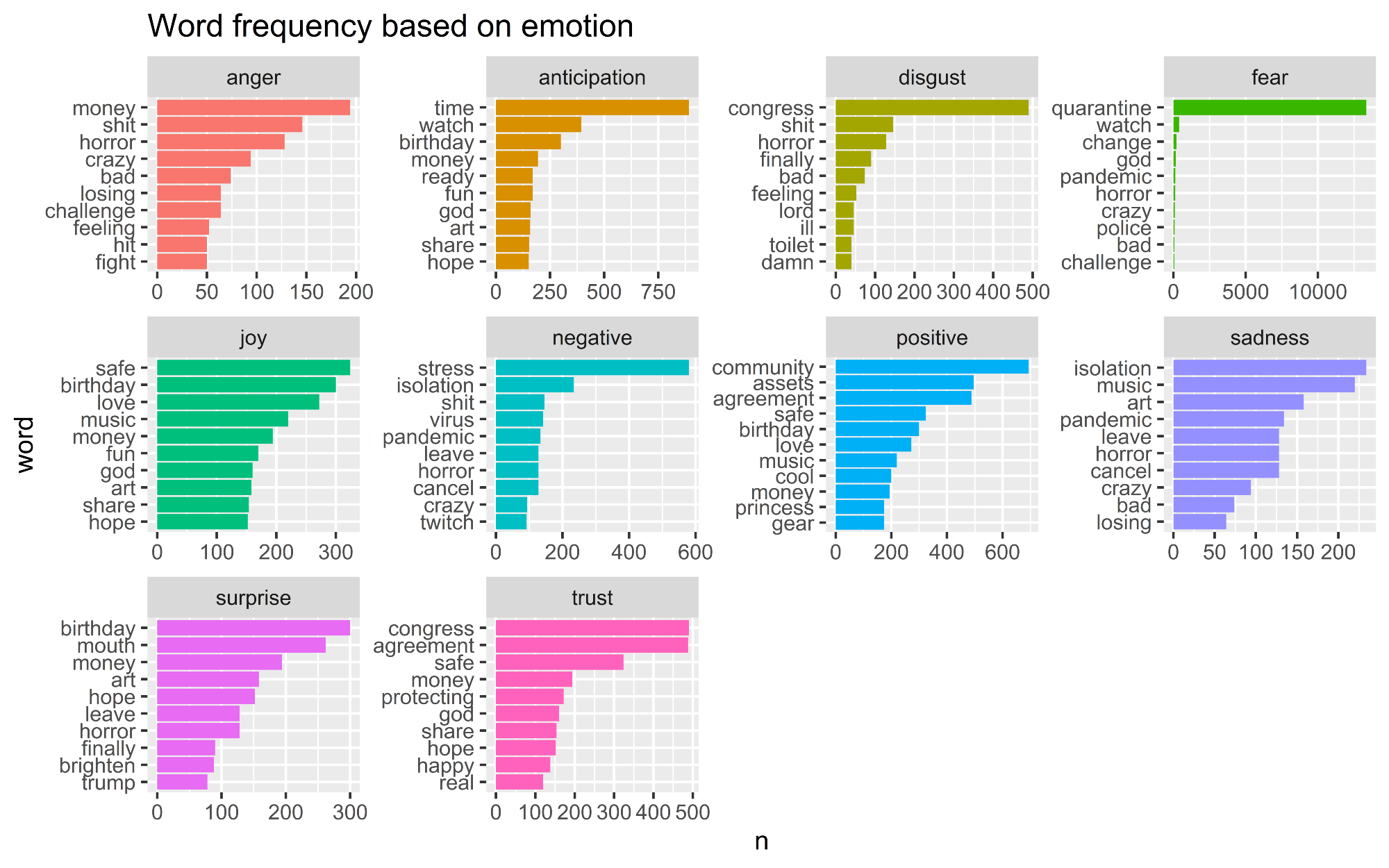`````` 在此期间，人们正在努力争取金钱，（没有）生日，音乐和艺术品
人们在谈论政府：国会与协议``````
``````#Ten types of emotion chart
tweets_sentiment <- no_stop_words %>%
inner_join(get_sentiments("nrc"), by = c("word" = "word"))tweets_sentiment %>%
count(word, sentiment, sort = TRUE)#ggplot
tweets_sentiment %>%
# Count by word and sentiment
count(word, sentiment) %>%
# Group by sentiment
group_by(sentiment) %>%
# Take the top 10 words for each sentiment
top_n(10) %>%
ungroup() %>%
mutate(word = reorder(word, n)) %>%
ggplot(aes(word, n, fill = sentiment)) +
geom_col(show.legend = FALSE) +
facet_wrap(~ sentiment, scales = "free") +
coord_flip() +
ggtitle("Word frequency based on emotion")``````

## 可视化单词关系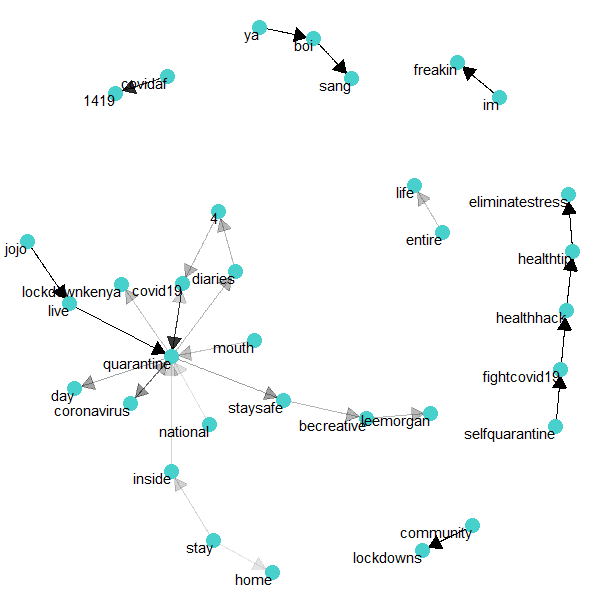“隔离”数据集的网络图

``````#Tokenize the dataset into bigrams
tweets_bigrams <- tweets %>%
select(id,
#       screenName,
text,
created) %>%
mutate(created_date = as.POSIXct(created, format="%m/%d/%Y %H")) %>%
mutate(text = replace_non_ascii(text, replacement = "", remove.nonconverted = TRUE)) %>%
mutate(text = str_replace_all(text, regex("@\\w+"),"" )) %>%
mutate(text = str_replace_all(text, regex("[[:punct:]]"),"" )) %>%
mutate(text = str_replace_all(text, regex("http\\w+"),"" )) %>%
unnest_tokens(bigram, text, token = "ngrams", n = 2)#Separate the bigrams into unigrams
bigrams_separated <- tweets_bigrams %>%
separate(bigram, c("word1", "word2"), sep = " ")#Remove stop words
bigrams_filtered <- bigrams_separated %>%
filter(!word1 %in% all_stop_words\$word) %>%
filter(!word2 %in% all_stop_words\$word)#Combine bigrams together
bigrams_united <- bigrams_filtered %>%
unite(bigram, word1, word2, sep = " ")``````

`````` 人们在隔离期间在推上写日记
在检疫期间，人们会听李·摩根（Lee Morgan）的爵士音乐
在检疫期间，Jojo的现场表演越来越受欢迎
自我隔离是与Covid-19对抗的一种方式，人们对健康技巧和消除压力的技巧很感兴趣``````

### 单词相关性分析—那么人们对社交距离的感觉如何？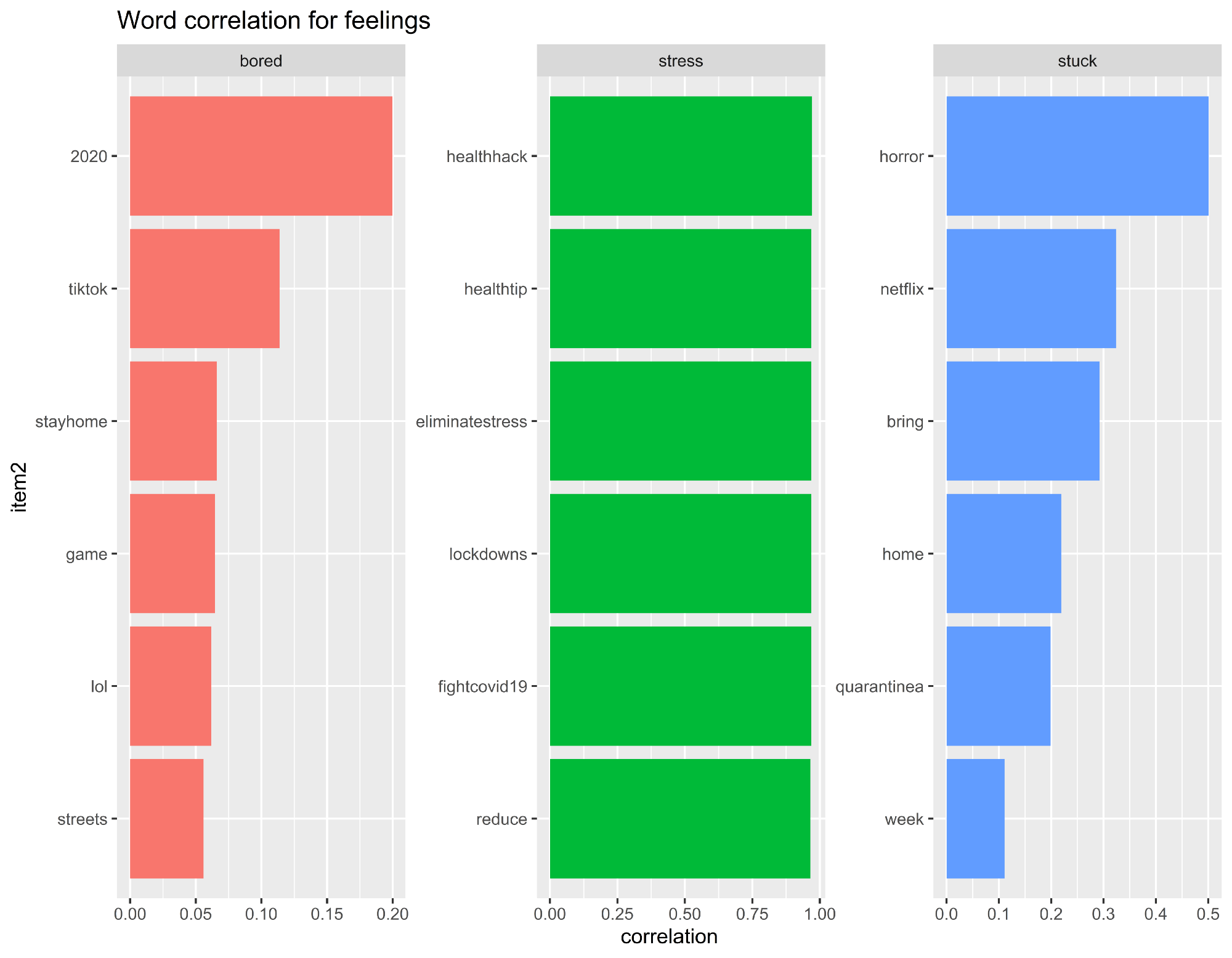`````` 人们在感到无聊时会使用TikTok（抖音的海外版）和游戏来消磨时间
乏味几乎可以概括大多数人在2020年的生活
造成压力，人们正在网上寻找减轻压力的提示
人们在家中“被困”时在Netflix上观看恐怖电影/连续剧``````

## 单词相关性分析—那么人们在家做什么呢？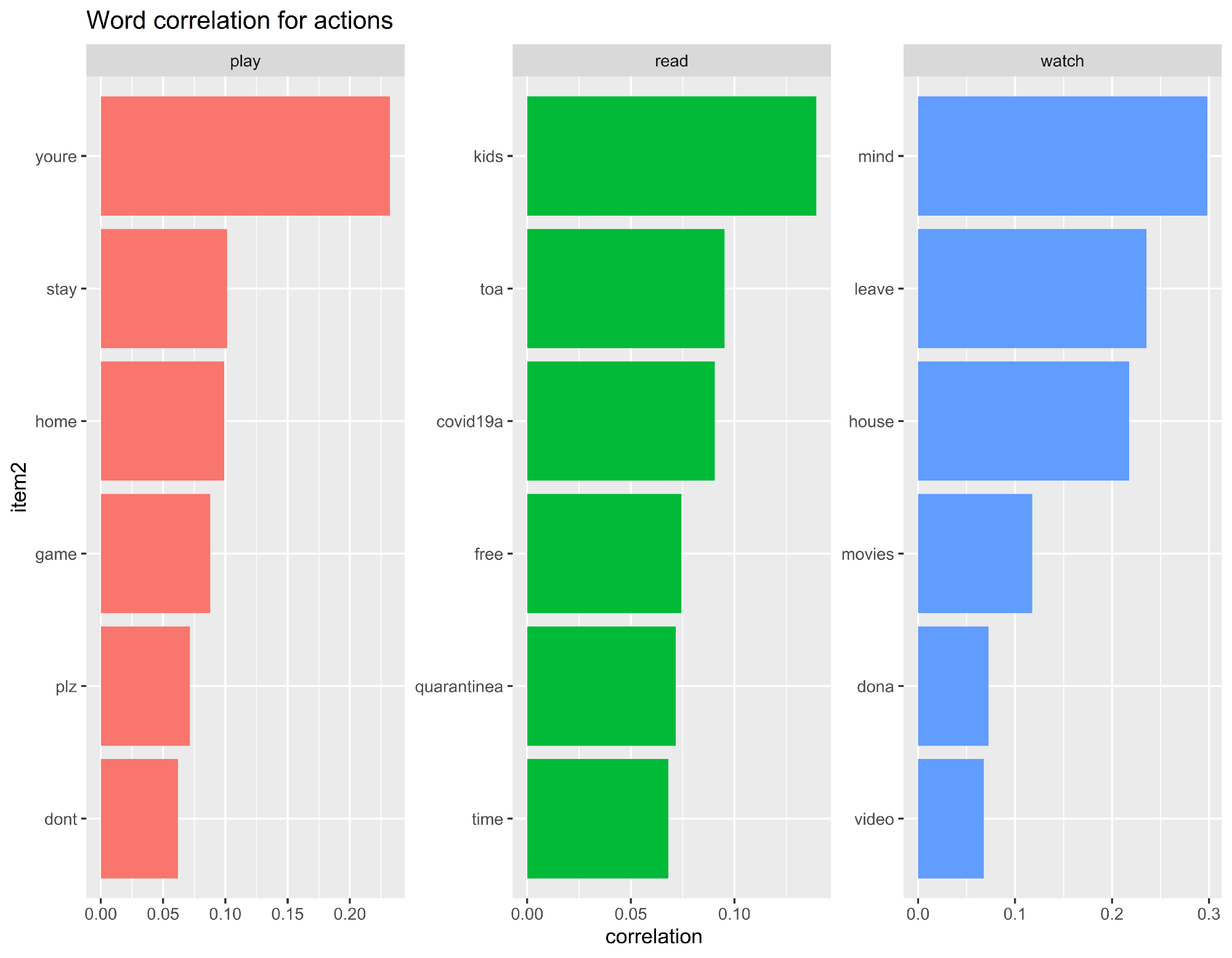`````` 大多数人可能会通过玩游戏，看电影和视频来度过自己的时间
人们花时间阅读他们的孩子
人们在此期间也终于有时间阅读``````

## 单词相关性分析-生日，金钱和社区…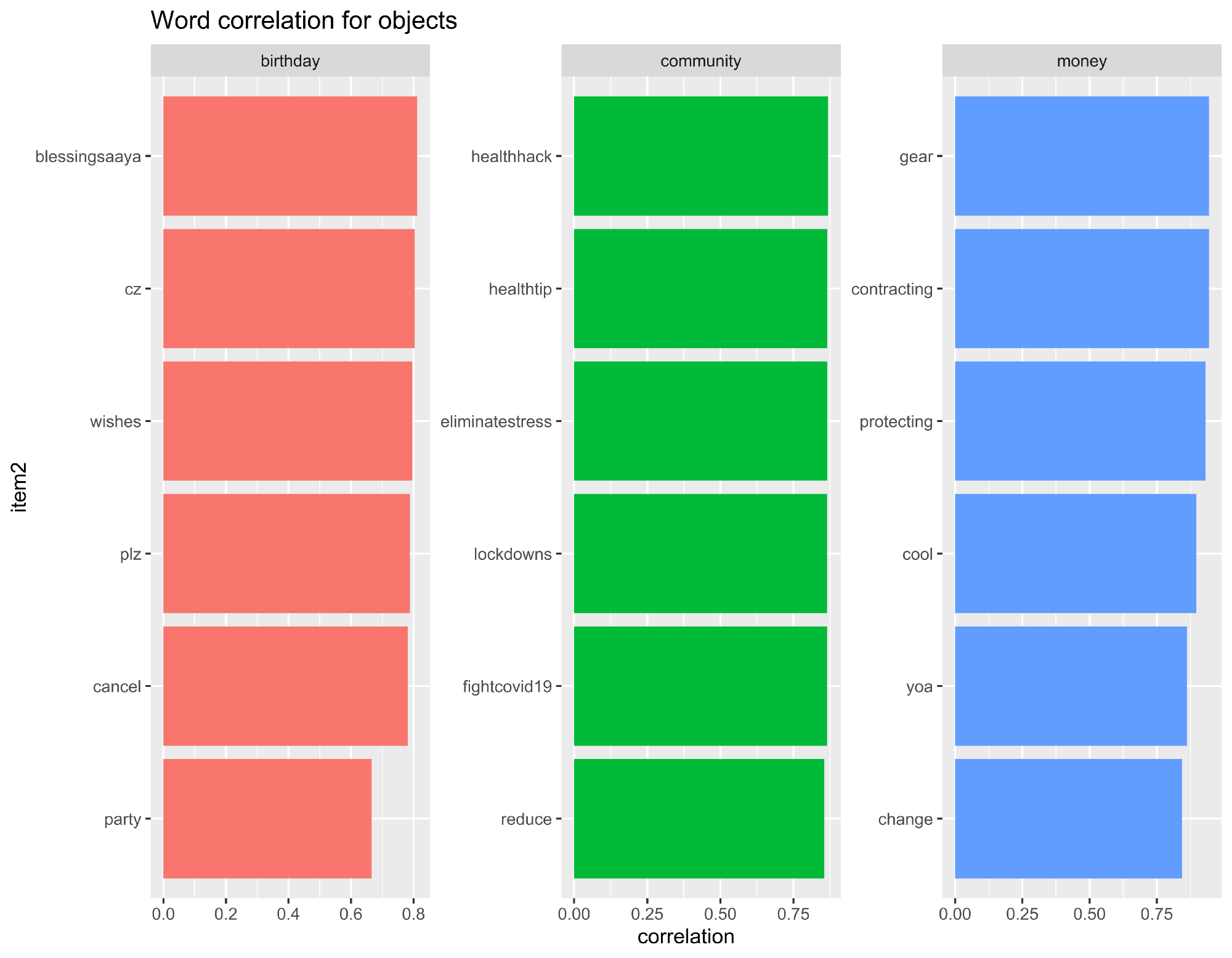`````` 生日聚会被取消。 取而代之的是，人们在推上表达自己的愿望
人们同意金钱并不能阻止我们感染该病毒的观点``````

## 结论

`````` 人们在冠状病毒情况下感到压力重重，但仍保持积极态度
在此居家和隔离期间，Tiktok和Netflix被广泛使用
人们将更多的时间花在与孩子，艺术，音乐和电影上``````

###### 评论